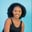Related Tags

python3
numpy

# How to use the np.true_divide() function for 2D arrays in PythonMaria Elijah

## Overview

The python library, NumPy, has a method called true_divide() which can be used to compute the true divide of two input arrays.

### The numpy.true_divide() method

The numpy.true_divide() method returns a true division, element-wise (element by element).

Note: A two-dimensional (2D) array can be created using a list of lists in Python.

### Syntax

numpy.true_divide(x1, x2, dtype=None, out=None)


### Parameters

• x1: This represents the dividend array.
• x2: This represents the divisor array.
• dtype: This is an optional parameter. It represents the return type of the array.
• out: This is an optional parameter. It represents the alternative output array in which the result is to be placed.

Note: If x1 and x2 have distinct shapes, they must be able to be broadcasted to a common shape for output representation.

### Return value

The numpy.true_divide() method returns scalar if the inputs are scalar. Otherwise, it returns an array (x1/x2).

Note: In Python, the operator / represents a true division. This is equivalent to the function true_divide().

### Code

The following code shows how to use the numpy.true_divide() method for two-dimensional (2D) arrays.

# import numpy
import numpy as np
# create a two 2D arrays
x1 = np.array([[2,6,5],[3,4,8]])
x2 = np.array([[1,7,2],[10,9,4]])

# divide the 2D arrays
# and store the result in result
result = np.true_divide(x1, x2)

print(result)
Implementation of np.true_divide() method

### Explanation

• Line 2: We import the numpy library.
• Lines 4–5: We create two 2D arrays called x1, and x2.
• Line 9: We use the np.true_divide() to divide the arrays x1 and x2. The result is stored in a new variable called result.
• Line 11: We display the result.

RELATED TAGS

python3
numpy

CONTRIBUTORMaria Elijah
RELATED COURSES

View all Courses

Keep Exploring

Learn in-demand tech skills in half the time